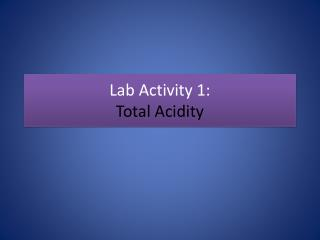# Lab Activity 1: Total Acidity - PowerPoint PPT PresentationDownload PresentationLab Activity 1: Total Acidity

Lab Activity 1: Total Acidity
Download Presentation## Lab Activity 1: Total Acidity

- - - - - - - - - - - - - - - - - - - - - - - - - - - E N D - - - - - - - - - - - - - - - - - - - - - - - - - - -
##### Presentation Transcript

1. Lab Activity 1:Total Acidity

2. Acidity depends on: • Acid strength in solution (measured by the degree of hydrogen ion (H+) dissociation 1. Strong acids: almost totally dissociates, releasing their hydrogen ions for bonding to other ions (HCl) 2. Weak acids: Have their H ions strong bonded (only few released) CH3COOH

3. Concentration of acidic compounds in solution. (expressed in: • %w/w, • %v/v, • ppm, • M (moles/l), • N (Equivalent Weight (EW/Volume)

4. Total Acidity • A measure of all the H+ of both dissociated and undissociated acids in solution. • Titratable acidity is used to approximate total acidity. • Titratable acidity can be measured by: 1- reacting the acid present with a base such as NaOH to the end point (equivalence point or neutrality point( at which H+ are equal to OH- in solution) indicated by a color change of an indicator.

5. Titrations • SA/SB –equivalence point is neutral. • WA/SB –equivalence point is basic. • WB/SA –equivalence point is acidic.

6. standard solution (in buret) unknown solution Titration • Titration • A standard solution with a known [ ] (the titrant) is used to determine the [ ] of an unknown solution. • The reaction that occurs is a neutralization reaction.

7. Titration • Equivalence point • Point in the rxn which equal amounts of H3O+ and OH- exist. • dramatic change in pH • End Point • Point at which a color change is observed. • The equivalence point and end point are not necessarily concurrent.

8. equivalence point OH- Na+ Na+ OH- OH- Na+ Na+ OH- H+ Cl Cl- H+ H+ Cl- H+ Cl- Titration Curve Titration of a Strong Acid With a Strong Base 14.0 12.0 Solution of NaOH Solution of NaOH 10.0 8.0 pH 6.0 4.0 Solution of HCl 2.0 0.0 0.0 10.0 20.0 30.0 40.0 Volume of 0.100 M NaOH added (mL)

9. Features of the Strong Acid-Strong Base Titration Curve • The pH starts out low, reflecting the high [H3O+] of the strong acid and increases gradually as acid is neutralized by the added base. • Suddenly the pH rises steeply. This occurs in the immediate vicinity of the equivalence point. For this type of titration the pH is 7.0 at the equivalence point. • Beyond this steep portion, the pH increases slowly as more base is added.

10. The four Major Differences Between a Strong Acid-Strong Base Titration Curve and a Weak Acid-Strong Base Titration Curve • The initial pH is higher. • A gradually rising portion of the curve, called the buffer region, appears before the steep rise to the equivalence point. • The pH at the equivalence point is greater than 7.00. • The steep rise interval is less pronounced.

11. Features of the Titration of a Polyprotic Acid with a Strong Base • The loss of each mole of H+ shows up as separate equivalence point (but only if the two pKas are separated by more than 3 pK units). • The pH at the midpoint of the buffer region is equal to the pKa of that acid species. • The same volume of added base is required to remove each mole of H+.

12. For Experimentsfollow the lab manual !!